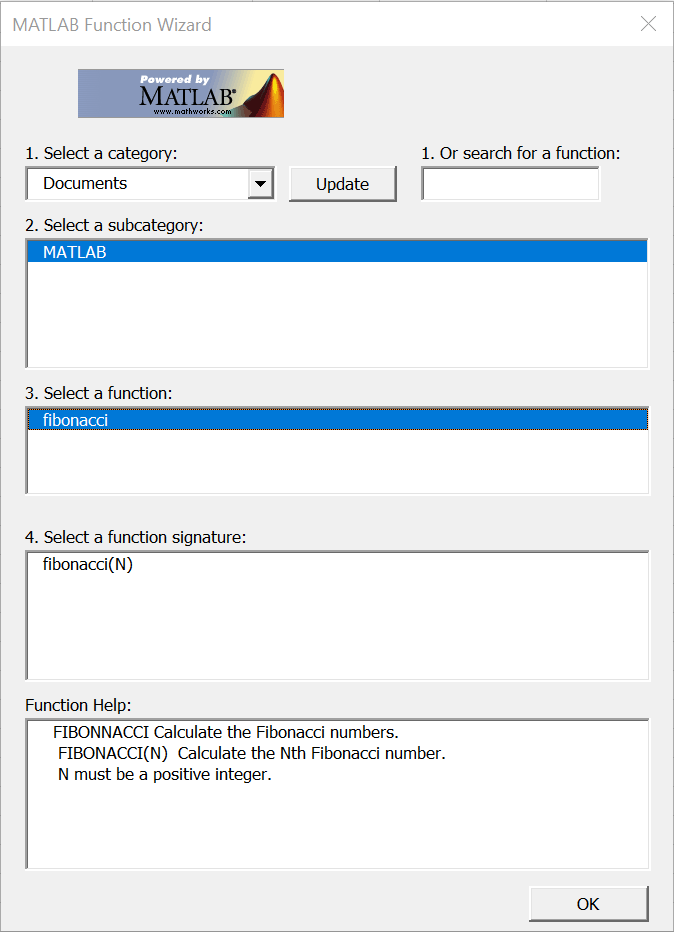Find Custom MATLAB Function Using MATLAB Function Wizard

This example shows how to write a custom function and find it using the MATLAB® Function Wizard for Spreadsheet Link™. To execute MATLAB functions using the MATLAB Function Wizard, see Find and Execute MATLAB Function Using MATLAB Function Wizard.

Create and save a custom function in MATLAB. First, create a help header in the function that contains supported function signatures to use with the MATLAB Function Wizard. Write the function that calculates the Fibonacci numbers, and then save the function in the folder Documents\MATLAB.

function f = fibonacci(n)
% FIBONACCI(N)  Calculate the Nth Fibonacci number.
% N must be a positive integer.
if n < 0
error('Invalid number.')
elseif n == 0
f = 0;
elseif n == 1
f = 1;
else
f = fibonacci(n - 1) + fibonacci(n - 2);
end;
end

For writing MATLAB functions, see Create Functions in Files.

Add the folder where you saved the function to the MATLAB search path. You can use the pathtool function or select Set Path in the MATLAB Toolstrip.

Open the MATLAB Function Wizard in Microsoft® Excel® using either the Microsoft Excel ribbon or context menu. Select the folder where you saved your function.To execute this function, follow the steps in Find and Execute MATLAB Function Using MATLAB Function Wizard.